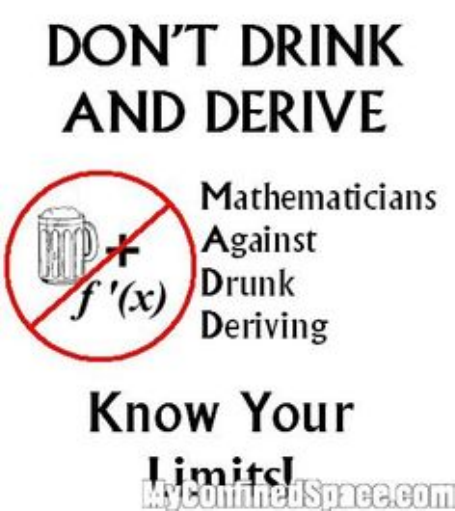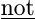Study Guides (380,000)
CA (150,000)
UOttawa (10,000)
MAT (600)
MAT 1300 (100)
Final

MAT 1300 Lecture 1: Curve sketchingExam

Department
Mathematics
Course Code
MAT 1300
Professor
Termeh Kousha
Study Guide
Final

This preview shows pages 1-3. to view the full 18 pages of the document.Curve Sketching
MAT 1300 A & D
Fall, 2016

Only pages 1-3 are available for preview. Some parts have been intentionally blurred.

1 THE SECOND-DERIVATIVE TEST 2
1 The Second-derivative Test
The Second-derivative Test: Let x=abe a critical number of a function
f(x) such that f(a) = 0.
1. If f′′(a)<0 then x=ais a relative maximum of f(x).
2. If f′′(a)>0 then x=ais a relative minimum of f(x).
Ex: Find and classify all the relative extrema of
f(x) = 3x4+ 16x330x29.

Only pages 1-3 are available for preview. Some parts have been intentionally blurred.1 THE SECOND-DERIVATIVE TEST 3
Ex: Use the second derivative test to ﬁnd and classify the critical points for
f(x) = (x28x+ 16)ex.
Note that just because f′′ (c) = 0 does not mean cis an inﬂection point.
Ex: show that f(x) = x4has a point cwhere the second derivative is zero
but x=cis not an inﬂection point.
You're Reading a Preview

Unlock to view full version